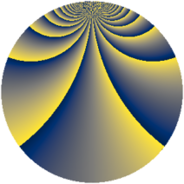# Properties

 Label 507.2.qLevel $507$ Weight $2$ Character orbit 507.q Rep. character $\chi_{507}(16,\cdot)$ Character field $\Q(\zeta_{39})$ Dimension $744$ Newform subspaces $2$ Sturm bound $121$ Trace bound $1$

# Related objects

## Defining parameters

 Level: $$N$$ $$=$$ $$507 = 3 \cdot 13^{2}$$ Weight: $$k$$ $$=$$ $$2$$ Character orbit: $$[\chi]$$ $$=$$ 507.q (of order $$39$$ and degree $$24$$) Character conductor: $$\operatorname{cond}(\chi)$$ $$=$$ $$169$$ Character field: $$\Q(\zeta_{39})$$ Newform subspaces: $$2$$ Sturm bound: $$121$$ Trace bound: $$1$$ Distinguishing $$T_p$$: $$2$$

## Dimensions

The following table gives the dimensions of various subspaces of $$M_{2}(507, [\chi])$$.

Total New Old
Modular forms 1512 744 768
Cusp forms 1416 744 672
Eisenstein series 96 0 96

## Trace form

 $$744q + 2q^{2} + q^{3} + 32q^{4} + 8q^{5} - q^{7} - 12q^{8} + 31q^{9} + O(q^{10})$$ $$744q + 2q^{2} + q^{3} + 32q^{4} + 8q^{5} - q^{7} - 12q^{8} + 31q^{9} + 2q^{10} - 6q^{11} - 4q^{12} - 34q^{13} + 24q^{14} - 2q^{15} + 26q^{16} - 10q^{17} - 4q^{18} + 2q^{20} + 10q^{21} - 64q^{22} - 6q^{23} + 12q^{24} - 46q^{25} - 30q^{26} - 2q^{27} - 18q^{28} - 8q^{29} + 4q^{30} - 62q^{31} - 116q^{32} - 2q^{33} - 134q^{34} - 10q^{35} + 32q^{36} + 12q^{37} - 82q^{38} + 6q^{39} - 50q^{40} + 10q^{41} + 248q^{42} - q^{43} + 16q^{44} - 4q^{45} - 4q^{46} - 12q^{47} - 4q^{48} - 140q^{49} - 28q^{50} - 8q^{51} - 100q^{52} - 128q^{53} - 134q^{55} - 40q^{56} - 24q^{57} - 78q^{58} + 402q^{59} - 104q^{60} + q^{61} - 150q^{62} - q^{63} - 44q^{64} - 4q^{65} - 96q^{66} - 153q^{67} - 136q^{68} + 6q^{69} - 200q^{70} + 190q^{71} + 6q^{72} + 2q^{73} - 196q^{74} - q^{75} + 56q^{76} + 28q^{77} - 102q^{78} + 2q^{79} + 22q^{80} + 31q^{81} - 132q^{82} + 48q^{83} - 14q^{84} - 142q^{85} - 156q^{86} - 106q^{87} + 484q^{88} + 4q^{89} - 4q^{90} - 135q^{91} + 24q^{92} - 3q^{93} - 170q^{94} + 168q^{95} - 8q^{96} - 171q^{97} + 22q^{98} + 12q^{99} + O(q^{100})$$

## Decomposition of $$S_{2}^{\mathrm{new}}(507, [\chi])$$ into newform subspaces

Label Dim. $$A$$ Field CM Traces $q$-expansion
$$a_2$$ $$a_3$$ $$a_5$$ $$a_7$$
507.2.q.a $$360$$ $$4.048$$ None $$1$$ $$-15$$ $$2$$ $$2$$
507.2.q.b $$384$$ $$4.048$$ None $$1$$ $$16$$ $$6$$ $$-3$$

## Decomposition of $$S_{2}^{\mathrm{old}}(507, [\chi])$$ into lower level spaces

$$S_{2}^{\mathrm{old}}(507, [\chi]) \cong$$ $$S_{2}^{\mathrm{new}}(169, [\chi])$$$$^{\oplus 2}$$